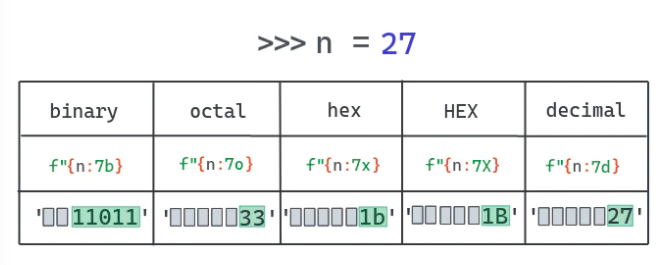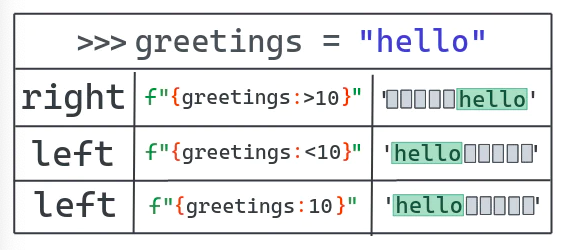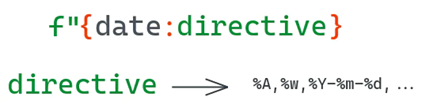Sec Hotspot 首页  排行榜  收藏本站  技术博客  RSS【漏洞预警】JumpServer 远程命令执行漏洞   2021.01.15 18:51:04  132美国防部又“拉黑”小米、中国商飞等9家中企(附名单)   2021.01.15 11:32:36  114为何incaseformat病毒在1月13日集中爆发,下次爆发时间为?   2021.01.15 18:00:05  96潜伏10+年,蠕虫病毒incaseformat今日作恶!   2021.01.13 21:20:22  93【安全风险通告】部分漏洞细节已公开,奇安信安全产品已支持防护,...   2021.01.20 12:24:56  80【漏洞通告】Laravel <= 8.4.2 _ignition 远程代码执行漏洞(CVE-...   2021.01.14 18:00:20  75干货 | 渗透之网站Getshell最全总结   2021.01.17 12:21:03  66工信部开展工业互联网企业网络安全分类分级管理试点工作   2021.01.14 16:14:05  62【安全风险通告】奇安信CERT监测到细节公开,JumpServer未授权访...   2021.01.16 00:42:35  59中文版!python版数据结构、机器学习、深度学习、TensorFlow和...   2021.01.16 08:10:35  57

f-string 竟然能有 73 个例子,我要学习下

（给 Python开发者 加星标，提升Python技能

1. f-string 是什么？

2. 用 python 做基本的文本格式化

3. f-string 局限性

4. 如何格式化表达式

5. 如何使用f-string调试你的代码

6. 如何在不同进制下格式化数字

7. 如何使用格式化字符串常量打印出对象

8. 如何在格式化字符串常量中设置浮点数精度

9. 如何将数字格式化为百分比

10. 如何调整或者增加f-string的缩进

11. 如何转义字符

12. 如何使字符串居中

13. 如何添加千位分隔符

13.1如何使用逗号作为千分位分隔符格式化数字

13.2如何使用逗号作为小数分隔符格式化数字

14.如何用科学计数法（指数计数法）格式化数字

15.在 f-string 中使用 if-else

16.如何在 f-string 中使用字典

17.如何用 f-string 拼接字符串

18.如何格式化 datetime 对象

19.如何改正 f-string 的无效语法错误

20.如何在字符串前补零

21.如何编写多行格式化字符串常量（怎样应对新行符号）

22.总结

1、python 中的 f-string 是什么？

``>>> msg = 'hello world'``>>> 'msg: %s' % msg``'msg: hello world'``

``>>> msg = 'hello world'``>>> 'msg: {}'.format(msg)``'msg: hello world'``

PEP 498 提出了一种新的字符串插值方法，该方法可以更简单便捷的使用 str.format 方法。你只需要在字符串开头加上一个字母 f，形成 f”” 的格式就可以了。

``>>> msg = 'hello world'``>>> f'msg: {msg}'``'msg: hello world'``

2、基本的字符串格式化``>>> book = "The dog guide"``>>> num_pages = 124``>>> f"The book {book} has{num_pages} pages"``'The book The dog guide has 124 pages'``>>> F"The book {book} has{num_pages} pages"``'The book The dog guide has 124 pages'``>>> print(Fr"The book {book} has{num_pages} pages\n")``The book The dog guide has 124 pages\n ``>>> print(FR"The book {book} has{num_pages} pages\n")``The book The dog guide has 124 pages\n``>>> print(f"The book {book} has{num_pages} pages\n")``The book The dog guide has 124 pages``

3、f-string 的限制

``>>> f"{name}"``---------------------------------------------------------------------------``NameError                                 Traceback(most recent call last)``<ipython-input-1-f0acc441190f> in<module>``----> 1 f"{name}"``NameError: name 'name' is not defined``

``>>> s = "{name}"``>>> s.format(name="Python")``'Python'``>>> print(s)``{name}``

``>>> f"My name is {name #name}!"``  File "<ipython-input-37-0ae1738dd871>", line 1``    f"My name is {name #name}!"``    ^``SyntaxError: f-string expression part cannot include '#'``

4、如何格式化一个表达式

``>>> f"4 * 4 is {4 * 4}"``'4 * 4 is 16'``

``>>> n = 4``>>> f"4 * 4 is {n * n}"``'4 * 4 is 16'``

5、如何使用 f-string 来调试代码

``>>> def magic_number():``     ...:     return 42``     ...: ``>>> f"{magic_number() = }"``'magic_number() = 42'``

6、如何格式化数字的不同进制f-string 还能在不同进制下显示数字。例如，你不需要通过b来对一个int进行格式转化就可以显示其二进制结果。

``>>> f'{7:b}'``'111'``

•  int 到二进制

•  int 到十六进制

•  int 到八进制

•  int 到十六进制(所有符号大写)

``>>> bases = {``       "b": "bin", ``       "o": "oct", ``       "x": "hex", ``       "X": "HEX", ``       "d": "decimal"``}``>>> for n in range(1, 21):``     ...:     for base, desc in bases.items():``     ...:         print(f"{n:5{base}}", end=' ')``     ...:     print()``    1     1     1     1     1 ``   10     2     2     2     2 ``   11     3     3     3     3 ``  100     4     4     4     4 ``  101     5     5     5     5 ``  110     6     6     6     6 ``  111     7     7     7     7 `` 1000    10     8     8     8 `` 1001    11     9     9     9 `` 1010    12     a     A    10 `` 1011    13     b     B    11 `` 1100    14     c     C    12 `` 1101    15     d     D    13 `` 1110    16     e     E    14 `` 1111    17     f     F    15 ``10000    20    10    10    16 ``10001    21    11    11    17 ``10010    22    12    12    18 ``10011    23    13    13    19 ``10100    24    14    14    20``

7、如何用 f-string 打印对象

• !r - 使用 repr() 将值转化为文本.

• !s - 使用 str() 将值转化为文本.

``>>> class Color:``    def __init__(self, r: float = 255, g: float = 255, b: float = 255):``        self.r = r``        self.g = g``        self.b = b``    def __str__(self) -> str:``        return "A RGB color"``    def __repr__(self) -> str:``        return f"Color(r={self.r}, g={self.g}, b={self.b})"``>>> c = Color(r=123, g=32, b=255)``# 如不加任何操作符, 会打印 __str__ 的值``>>> f"{c}"``'A RGB color'``# 用`obj!r` 的话会打印 __repr__ 的值``>>> f"{c!r}"``'Color(r=123, g=32, b=255)'``# 使用!s跟默认值一样``>>> f"{c!s}"``'A RGB color'``

Python也允许通过定义不同类型使用__format__方法控制格式化结果，下面的例子会展示所有可能情况。

``>>> class Color:``    def __init__(self, r: float = 255, g: float = 255, b: float = 255):``        self.r = r``        self.g = g``        self.b = b``    def __str__(self) -> str:``        return "A RGB color"``    def __repr__(self) -> str:``        return f"Color(r={self.r}, g={self.g}, b={self.b})"``>>> c = Color(r=123, g=32, b=255)``# When no option is passed, the __str__ result is printed``>>> f"{c}"``'A RGB color'``# When `obj!r` is used, the __repr__ output is printed``>>> f"{c!r}"``'Color(r=123, g=32, b=255)'``# Same as the default``>>> f"{c!s}"``'A RGB color'``Python also allows us to control the formatting on a per-type basis through the __format__ method. The following example shows how you can do all of that.``>>> class Color:``    def __init__(self, r: float = 255, g: float = 255, b: float = 255):``        self.r = r``        self.g = g``        self.b = b``    def __str__(self) -> str:``        return "A RGB color"``    def __repr__(self) -> str:``        return f"Color(r={self.r}, g={self.g}, b={self.b})"``    def __format__(self, format_spec: str) -> str:``        if not format_spec or format_spec == "s":``            return str(self)``        if format_spec == "r":``            return repr(self)``        if format_spec == "v":``            return f"Color(r={self.r}, g={self.g}, b={self.b}) - A nice RGB thing."``        if format_spec == "vv":``            return (``                f"Color(r={self.r}, g={self.g}, b={self.b}) "``                f"- A more verbose nice RGB thing."``            )``        if format_spec == "vvv":``            return (``                f"Color(r={self.r}, g={self.g}, b={self.b}) "``                f"- A SUPER verbose nice RGB thing."``            )``        raise ValueError(``            f"Unknown format code '{format_spec}' " "for object of type 'Color'"``        )``>>> c = Color(r=123, g=32, b=255)``>>> f'{c:v}'``'Color(r=123, g=32, b=255) - A nice RGB thing.'``>>> f'{c:vv}'``'Color(r=123, g=32, b=255) - A more verbose nice RGB thing.'``>>> f'{c:vvv}'``'Color(r=123, g=32, b=255) - A SUPER verbose nice RGB thing.'``>>> f'{c}'``'A RGB color'``>>> f'{c:s}'``'A RGB color'``>>> f'{c:r}'``'Color(r=123, g=32, b=255)'``>>> f'{c:j}'``---------------------------------------------------------------------------``ValueError                                Traceback (most recent call last)``<ipython-input-20-1c0ee8dd74be> in <module>``----> 1 f'{c:j}'``<ipython-input-15-985c4992e957> in __format__(self, format_spec)``     29                 f"- A SUPER verbose nice RGB thing."``     30             )``---> 31         raise ValueError(``     32             f"Unknown format code '{format_spec}' " "for object of type 'Color'"``     33         )``ValueError: Unknown format code 'j' for object of type 'Color'``

docs.python.org/3/library/functions.html#as..

``>>> utf_str = "Áeiöu"``>>> f"{utf_str!a}"``"'\\xc1ei\\xf6u'"``

8、如何用f-string设定浮点数精度

F-string可以像str.format那样格式化浮点数。想要实现这一点，你需要加一个 : （冒号）再加一个 . （英文句号）然后跟着小数点位数最后以f结尾。

``>>> num =4.123956``>>> f"num rounded to 2 decimalpalces = {num:.2f}"``'num rounded to 2 decimal palces = 4.12’``

``>>> print(f'{num}')``4.123956``

9、如何将一个数字格式化为百分数

Python f-string方法有个非常便捷的实现格式化百分数的操作方法。方法与浮点数格式化类似，但是要用%代替结尾的f。它会将原始数值乘以100并显示成有百分符号的固定格式。精度一样也是可以设定的。

``>>> total = 87``>>> true_pos = 34``>>> perc = true_pos / total``>>> perc``0.39080459770114945``>>> f"Percentage of true positive: {perc:%}"``'Percentage of true positive: 39.080460%'``>>> f"Percentage of true positive: {perc:.2%}"``'Percentage of true positive: 39.08%'``

10、如何调整或者增加 f-string 的缩进``>>> greetings = "hello"``>>> f"She says {greetings:>10}"``'She says      hello'``# Pad 10 char to the right``>>> f"{greetings:>10}"``'     hello'``>>> f"{greetings:<10}"``'hello     '``# You can omit the < for left padding``>>> f"{greetings:10}"``'hello     '````>>> a = "1"``>>> b = "21"``>>> c = "321"``>>> d = "4321"``>>> print("\n".join((f"{a:>10}", f"{b:>10}", f"{c:>10}", f"{d:>10}")))``         1``        21``       321``      4321``

11、如何转义符号

``>>> hello = "world"``>>>f"{{hello}} = {hello}"``'{hello} = world'``

``>>>f"{hello} = \"hello\""``'world = "hello"'``

12、如何使字符串居中``>>> hello = "world"``>>>f"{hello:^11}"``'  world   '``>>>f"{hello:*^11}"``'***world***'``# Extra padding is added to the right``>>>f"{hello:*^10}"``'**world***'``# N shorter than len(hello)``>>>f"{hello:^2}"``'world'``

13、如何格式化千分位F-string也允许我们自定义数字显示的格式。有个非常普遍的需求就是把数字以每3位为一个间隔使用下划线进行分隔。

``>>> big_num = 1234567890``>>> f"{big_num:_}"``'1_234_567_890'``

13.1  如何使用逗号千分位分隔符数字

``>>> big_num = 1234567890``>>> f"{big_num:,}"``'1,234,567,890'``

``>>> num =2343552.6516251625``>>> f"{num:,.3f}"``‘2,343,552.652'``

13.2 如何用空格做千分位分隔符

``>>> big_num = 1234567890``>>> f"{big_num:,}".replace(',',' ')``'1 234 567 890'``

https://stackoverflow.com/a/17484665

14、如何用科学计数法（指数计数法）显示一个数字

``>>> num =2343552.6516251625``>>>f"{num:e}"``'2.343553e+06'``>>> f"{num:E}"``'2.343553E+06'``>>> f"{num:.2e}"``'2.34e+06'``>>> f"{num:.4E}"``'2.3436E+06'``

15、在 f-string 中使用 if-else

F-string也能计算稍微复杂的运算式，比如if/else

``>>> a = "this is a"``>>> b = "this is b"``>>> f"{a if 10 > 5 else b}"``'this is a'``>>> f"{a if 10 < 5 else b}"``'this is b'``

16、如何在 f-string 中使用字典

``>>>color = {"R": 123, "G": 145, "B": 255}``>>> f"{color['R']}"``'123'``>>> f'{color["R"]}'``‘’123'此处有误啊应该是‘123’吧``>>> f"RGB = ({color['R']},{color['G']}, {color['B']})"``'RGB = (123, 145, 255)’``

17、如何用 f-string 拼接字符串

``# 隐式字符串拼接``>>> f"{123}" " = "f"{100}" " + " f"{20}" " + "f"{3}"``'123 = 100 + 20 + 3'``# 使用加号 + 的显式字符串拼接``>>> f"{12}" + " != "+ f"{13}"``'12 != 13'``# 使用str.join的字符串拼接``>>> "".join((f"{13}", f"{45}"))``'13 45'``>>>"#".join((f"{13}", f"{45}"))``'13#45'``

18、如何格式化 datetime 对象

F-string也支持datetime对象的格式化。其过程与str.format格式化日期的方法很近似。请查阅官方文档中的表格获取更多所支持格式的相关信息。``>>> import datetime``>>> now = datetime.datetime.now()``>>> ten_days_ago = now -datetime.timedelta(days=10)``>>> f'{ten_days_ago:%Y-%m-%d %H:%M:%S}'``'2020-10-13 20:24:17'``>>> f'{now:%Y-%m-%d %H:%M:%S}'``'2020-10-23 20:24:17'``

19、如何修复f-string的非法格式错误

``>>>color = {"R": 123, "G": 145, "B": 255}``>>> f"{color["R"]}"``  File"<ipython-input-43-1a7f5d512400>", line 1``   f"{color["R"]}"``   ^``SyntaxError: f-string: unmatched '['``>>> f'{color['R']}'``  File"<ipython-input-44-3499a4e3120c>", line 1``   f'{color['R']}'``   ^``SyntaxError: f-string: unmatched '['``

``>>> f"this is an old version"`` File"<stdin>", line 1``   f"this is an old version"``SyntaxError: invalid syntax``

``>>> import sys;print(sys.version)``2.7.18 (default, Apr 202020, 19:27:10)``[GCC 8.3.0]``

20、如何在字符串前补零

https://docs.python.org/3/library/string.html#format-specification-mini-language

``>>> num = 42``>>> f"{num:05}"``'00042'``>>> f'{num:+010}'``'+000000042'``>>> f'{num:-010}'``'0000000042'``>>> f"{num:010}"``'0000000042'``>>> num = -42``>>> f'{num:+010}'``'-000000042'``>>> f'{num:010}'``'-000000042'``>>> f'{num:-010}'``'-000000042'``

21、如何处理多行f-string（换行符的处理）

``>>> multi_line = (f'R: {color["R"]}\nG: {color["G"]}\nB: {color["B"``    ...: ]}\n')``>>> multi_line``'R: 123\nG: 145\nB: 255\n'``>>> print(multi_line)``R: 123``G: 145``B: 255``

``>>> other = f"""R:{color["R"]}``    ...: G:{color["G"]}``    ...: B:{color["B"]}``    ...:"""``>>> print(other)``R: 123``G: 145``B: 255``

``>>> other = f'''``    ...:this is an example``    ...:``    ...:^Iof color {color["R"]}``   ...:    ``    ...: '''``>>> other``'\nthis is an example\n\n\tof color 123\n    \n'``>>> print(other)``this is an example``    of color123``>>>``

22、结论

- EOF -